Publicité

# Linear equations rev - copy

15 Jul 2011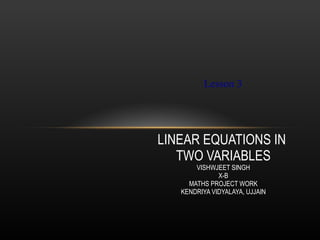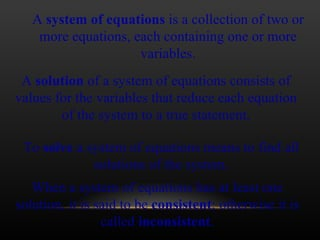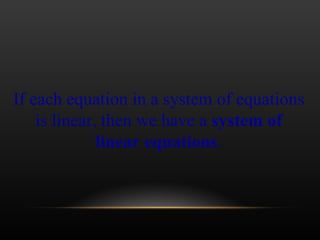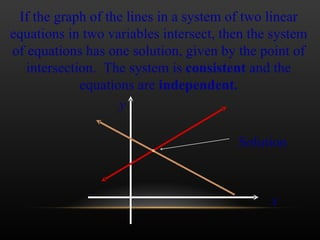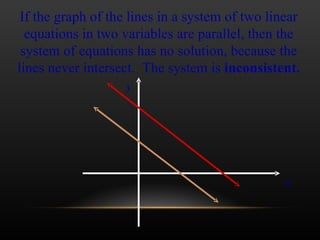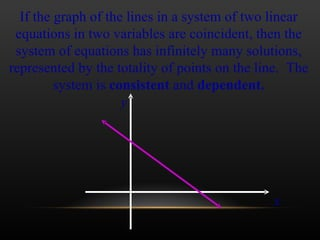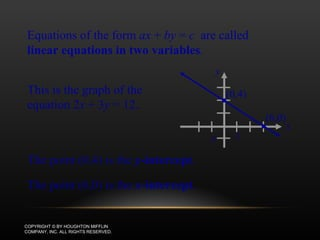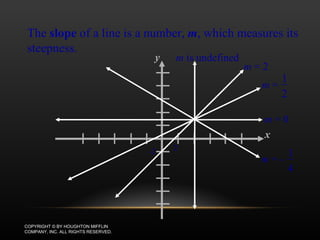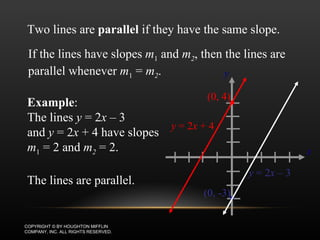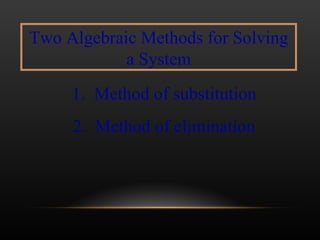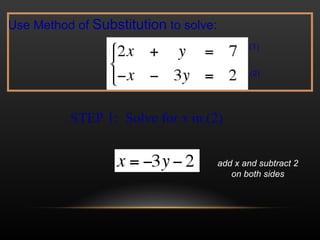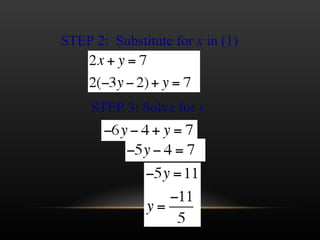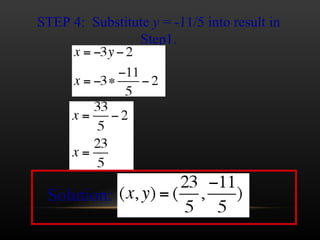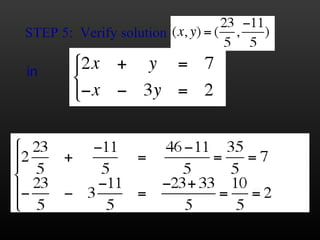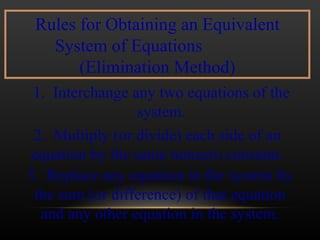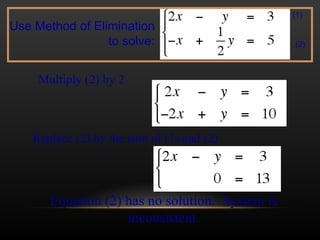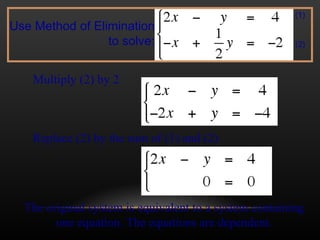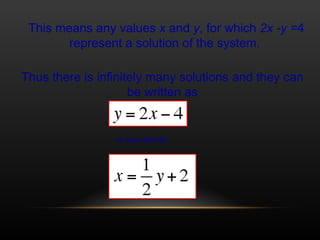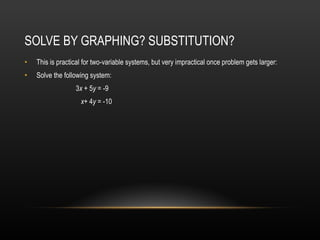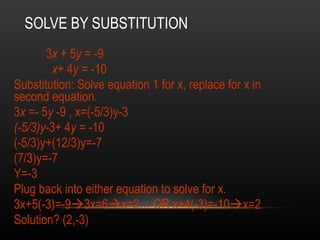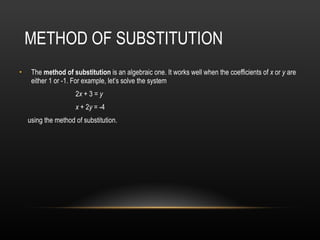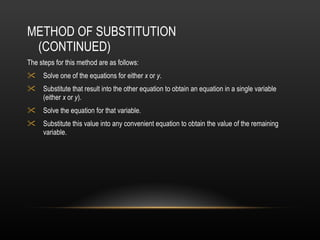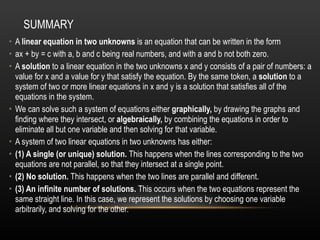1 sur 23
Publicité

### Linear equations rev - copy

1. LINEAR EQUATIONS IN TWO VARIABLES VISHWJEET SINGH X-B MATHS PROJECT WORK KENDRIYA VIDYALAYA, UJJAIN Lesson 3
2. A system of equations is a collection of two or more equations, each containing one or more variables. A solution of a system of equations consists of values for the variables that reduce each equation of the system to a true statement. When a system of equations has at least one solution, it is said to be consistent ; otherwise it is called inconsistent . To solve a system of equations means to find all solutions of the system.
3. If each equation in a system of equations is linear, then we have a system of linear equations .
4. If the graph of the lines in a system of two linear equations in two variables intersect, then the system of equations has one solution, given by the point of intersection. The system is consistent and the equations are independent. Solution y x
5. If the graph of the lines in a system of two linear equations in two variables are parallel, then the system of equations has no solution, because the lines never intersect. The system is inconsistent. x y
6. If the graph of the lines in a system of two linear equations in two variables are coincident, then the system of equations has infinitely many solutions, represented by the totality of points on the line. The system is consistent and dependent. x y
7. LINEAR EQUATIONS COPYRIGHT © BY HOUGHTON MIFFLIN COMPANY, INC. ALL RIGHTS RESERVED. Equations of the form ax + by = c are called linear equations in two variables . The point (0,4) is the y -intercept . The point (6,0) is the x -intercept . x y 2 -2 This is the graph of the equation 2 x + 3 y = 12. (0,4) (6,0)
8. SLOPE OF A LINE COPYRIGHT © BY HOUGHTON MIFFLIN COMPANY, INC. ALL RIGHTS RESERVED. The slope of a line is a number, m , which measures its steepness. m = 0 m = 2 m is undefined y x 2 -2 m = 1 2 m = - 1 4
9. EXAMPLE: PARALLEL LINES COPYRIGHT © BY HOUGHTON MIFFLIN COMPANY, INC. ALL RIGHTS RESERVED. Two lines are parallel if they have the same slope. If the lines have slopes m 1 and m 2 , then the lines are parallel whenever m 1 = m 2 . Example : The lines y = 2 x – 3 and y = 2 x + 4 have slopes m 1 = 2 and m 2 = 2. The lines are parallel. x y y = 2 x + 4 (0, 4) y = 2 x – 3 (0, -3)
10. Two Algebraic Methods for Solving a System 1. Method of substitution 2. Method of elimination
11. STEP 1: Solve for x in (2) Use Method of Substitution to solve: (1) (2) add x and subtract 2 on both sides
12. STEP 2: Substitute for x in (1) STEP 3: Solve for y
13. STEP 4: Substitute y = -11/5 into result in Step1. Solution:
14. STEP 5: Verify solution in
15. Rules for Obtaining an Equivalent System of Equations (Elimination Method) 1. Interchange any two equations of the system. 2. Multiply (or divide) each side of an equation by the same nonzero constant. 3. Replace any equation in the system by the sum (or difference) of that equation and any other equation in the system.
16. Multiply (2) by 2 Replace (2) by the sum of (1) and (2) Equation (2) has no solution. System is inconsistent. Use Method of Elimination to solve: (1) (2)
17. Multiply (2) by 2 Replace (2) by the sum of (1) and (2) The original system is equivalent to a system containing one equation. The equations are dependent. Use Method of Elimination to solve: (1) (2)
18. This means any values x and y , for which 2x -y = 4 represent a solution of the system. Thus there is infinitely many solutions and they can be written as or equivalently
Publicité